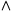The tricky part is:

5) Calculate the constraints of the program C, and the properties we're checking of the program P.

For a program I, the constraints are C(I,true) where C(I,g) is defined:

C(I;J, g) = C(I, g)C(I, g)
C(if (c) { I } else { J }, g) = C(I, gc)C(J, gc)
C(assume(c), g) = gc
C(x=e, g) = gx=e
C(anything else, g) = true

For a program I, the properties are P(I,true) where P(I,g) is defined:

P(I;J, g) = P(I, g)P(I, g)
P(if (c) { I } else { J }, g) = P(I, gc)P(J, gc)
P(assert(c), g) = gc
P(anything else, g) = true